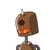# 18 ] Divide 20 chocolates between Lily and Kim in theratio 3:21. lily = 15 chocolates, kim = 9 chocolates2. lily

18 ] Divide 20 chocolates between Lily and Kim in the
ratio 3:2
1. lily = 15 chocolates, kim = 9 chocolates
2. lily = 6 chocolates, kim 5 chocolates
3. lily = 12 chocolates, kim = 8 chocolates
4. none​

1.3rd is the answer 12 for lily and 8 for kim.

12:8 = 3:2

Step-by-step explanation:

1. 15:9=15/9

= 3:5

2. 6:5 = 6/5

= 1.2:1

3. 12:8 = 12/8

= 3:2